Objectives: :

• Define arithmetic sequences and series.
• Use of the formulae for the nth term and the sum of the first n terms of the sequence.
• Use of sigma notation for sums of arithmetic sequences.
##### Votre titre va ici

Your content goes here. Edit or remove this text inline or in the module Content settings. You can also style every aspect of this content in the module Design settings and even apply custom CSS to this text in the module Advanced settings.

## SEQUENCE

### A sequence is just an ordered list of numbers (terms in a definite order). For example :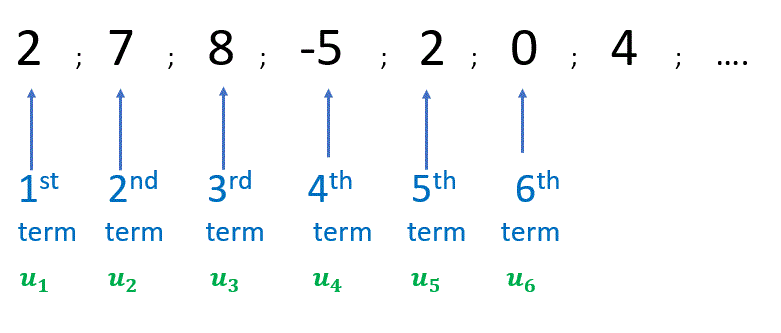## SIGMA NOTATION $$\sum\limits_{n=1}^{k}$$

### Examples :## Definition:

### Arithmetic sequences are characterized by the fact that to get from one term to the next we always add the same amount.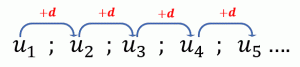### The amount we add is known as the common difference and is usually referred to as d, $$d \in \mathbb{R}$$

For example :

The sequence whose first few terms are: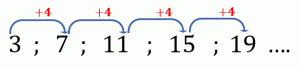is arithmetic, with common difference $$d=4$$

### The common difference $$d$$

Examples :

If $$u_1 =1, d=2$$ the sequence is $$1 ; 3 ; 5 ; 7 ; 9 ; …$$

If $$u_1 =-10, d=5$$ the sequence is $$-10 ; -5 ; 0 ; 5 ; 10 ; …$$

If $$u_1 =10, d=-3$$ the sequence is $$10 ; 7 ; 4 ; 1 ; -2 ; …$$What is the general formula for $$u_n$$ ?

Let us think:

In order to find $$u_3$$ , we start from $$u_1$$ and then add 2 times the difference $$d$$. $$u_{\color{orange}{3}}=u_1+\color{orange}{2}d$$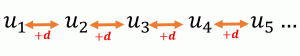Hence,

$$u_{\color{orange}{4}} = u_1+\color{orange}{ 3}d$$

$$u_{\color{orange}{5}} = u_1+ \color{orange}{4}d$$

Similarly,

$$u_{\color{orange}{10}} = u_1+ \color{orange}{9}d$$

$$u_{\color{orange}{50}} = u_1+ \color{orange}{49}d$$

In general,

$$u_n = u_1+ (n-1)d$$

### where $$a_1$$ is the first term and $$d$$ the common difference.

##### 2. Sum of an arithmetic sequenceGauss Problem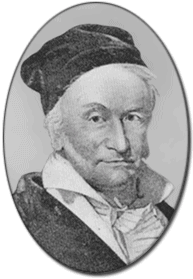In elementary school in the late 1700’s, Gauss was asked to find the sum of the numbers from 1 to 100.  The question was assigned as “busy work” by the teacher, but Gauss found the answer rather quickly by discovering a pattern. $$1+2+3+4+5+6+ ….+97+98+99+100 = ?$$

The answer is 5050. How can you do it without a calculator in a matter of minutes like Gauss?

### $$d$$, the common difference.

##### Teachers Ressources

Quizizz as a warm up or at the end of your course. This resource can be used by the students themselves by clicking on the “practice” button:

 – test